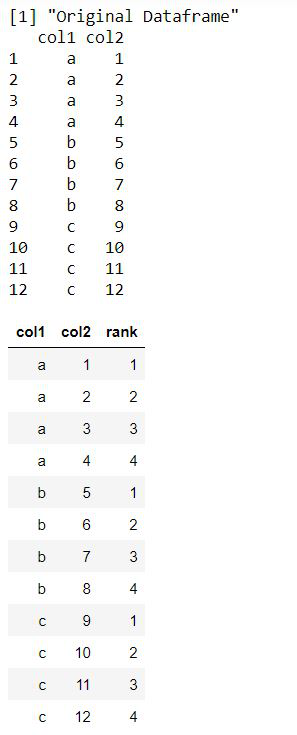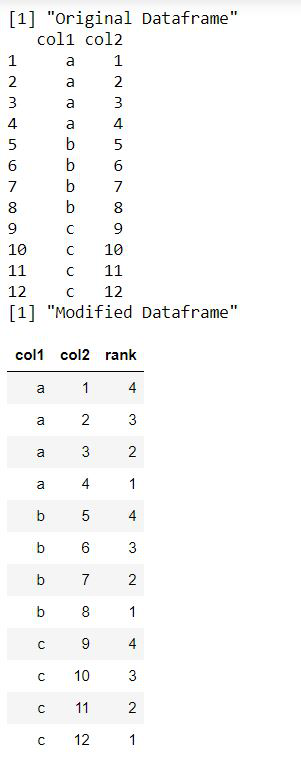Open in App
Not now

# Rank variable by group using Dplyr package in R

• Difficulty Level : Expert
• Last Updated : 17 Oct, 2021

In this article, we are going to see how to rank the variable by group using dplyr in R Programming Language.

The dplyr package in R is used to perform mutations and data manipulations in R. It is particularly useful for working with data frames and data tables. The package can be downloaded and installed into the working directory using the following command :

`install.packages("dplyr")`

## Arranging by rank in ascending order

The arrange() method is invoked to arrange the data of the data frame in the ascending order or descending order. It is used to reorder the rows of the data frame based on the variable names.

`arrange(col-name)`

This is followed by the application of the group_by method which takes as arguments the set of column names that are used for grouping the data. It may comprise one or more columns.

`group_by(col-name1, col-name2..)`

The mutate() method can then be applied to the data frame to perform manipulations or modifications by changing the orientation of the data stored in the data frame. The rank() method is used to assign numerical rankings to the data values mapped to the groups assigned. By default, the rank is displayed in ascending order.

`rank(col-name)`

Code:

## R

 `library``(``"dplyr"``)`` ` `# creating a data frame``data_frame <- ``data.frame``(col1 = ``rep``(``letters``[1:3],each = 4),``                          ``col2 = 1:12)`` ` `print ``(``"Original Dataframe"``)``print ``(data_frame)``data_frame %>% ``arrange``(col1, col2) %>%``  ``group_by``(col1) %>% ``  ``mutate``(rank = ``rank``(col2))`

Output:## Arranging by rank in descending order

In order to display the ranks in descending order, the col name is prepended with a minus sign. This displays the numerical ranks of the vector in decreasing order. The new column name can be assigned to the output of this method. The output data frame contains an additional column with the same name as that of the assigned name.

## R

 `library``(``"dplyr"``)`` ` `# creating a data frame``data_frame <- ``data.frame``(col1 = ``rep``(``letters``[1:3],each = 4),``                          ``col2 = 1:12)`` ` `print ``(``"Original Dataframe"``)``print ``(data_frame)`` ` `# ranking by col1 variable``print ``(``"Modified Dataframe"``)`` ` `# ranking in descending order``data_frame %>% ``arrange``(col1, col2) %>%``  ``group_by``(col1) %>% ``  ``mutate``(rank = ``rank``(-col2))`

Output:My Personal Notes arrow_drop_up